06 Oct
06Oct

Author: Kevin Gildea

We can integrate kinematic differential equations to determine both 1) where an object will be and 2) what orientation it will have after a specified amount of time. In this post I describe the mathematical procedure, and implement the solution in Python.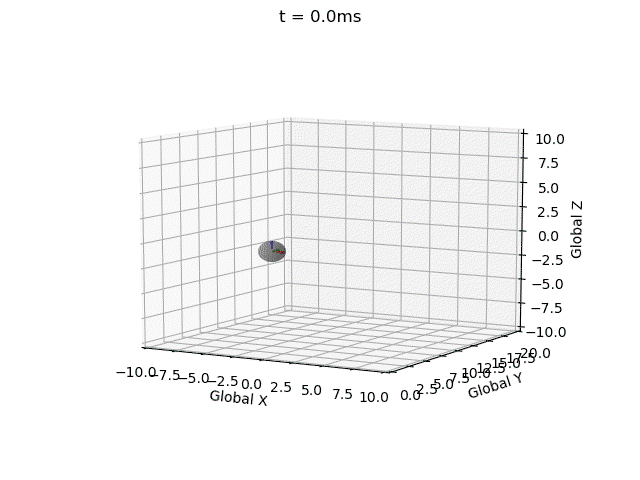### Euler Integration

Euler integration is a 1st order initial value numerical procedure for solving ordinary differential equations (ODEs). The method can be used to approximate the position/orientation of an object after a period of time, given the initial degrees of freedom, and their 1st derivatives (i.e. dynamics are not considered). The error in the approximation is proportional to the size of the time step used. For our problem we would like to determine both orientation and position, where angular velocity and linear velocity are specified (their 1st derivatives).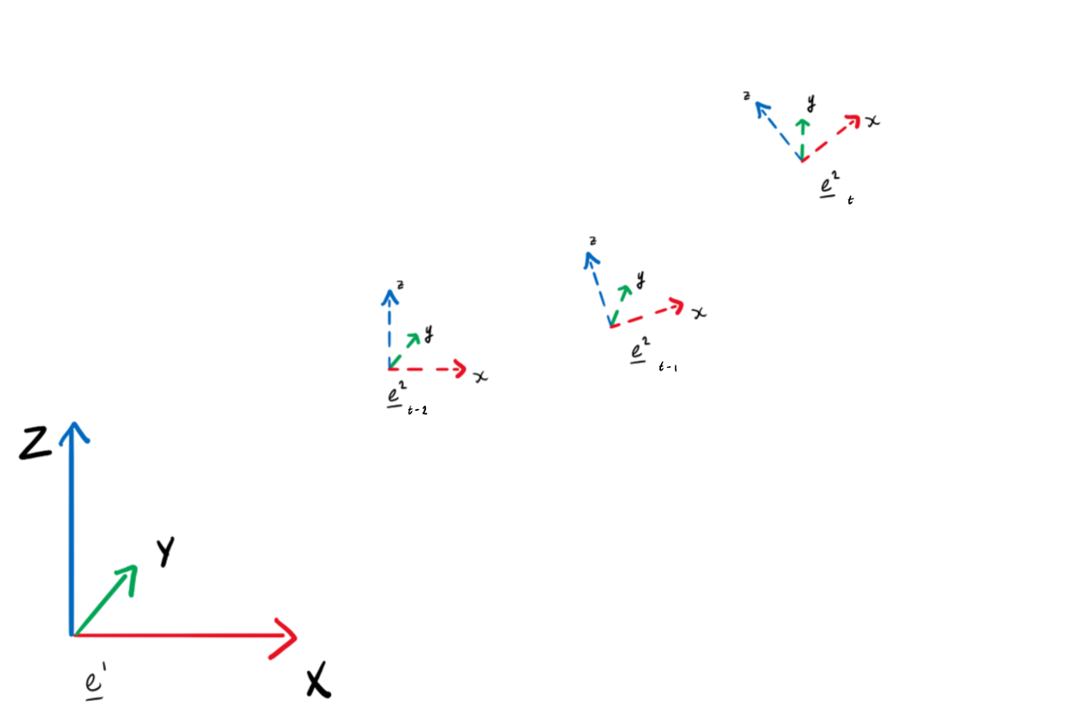The Euler integration equation for the orientation is apparent, however, the relationship between the angular velocity vector and the 1st time derivative of the rotation matrix is not.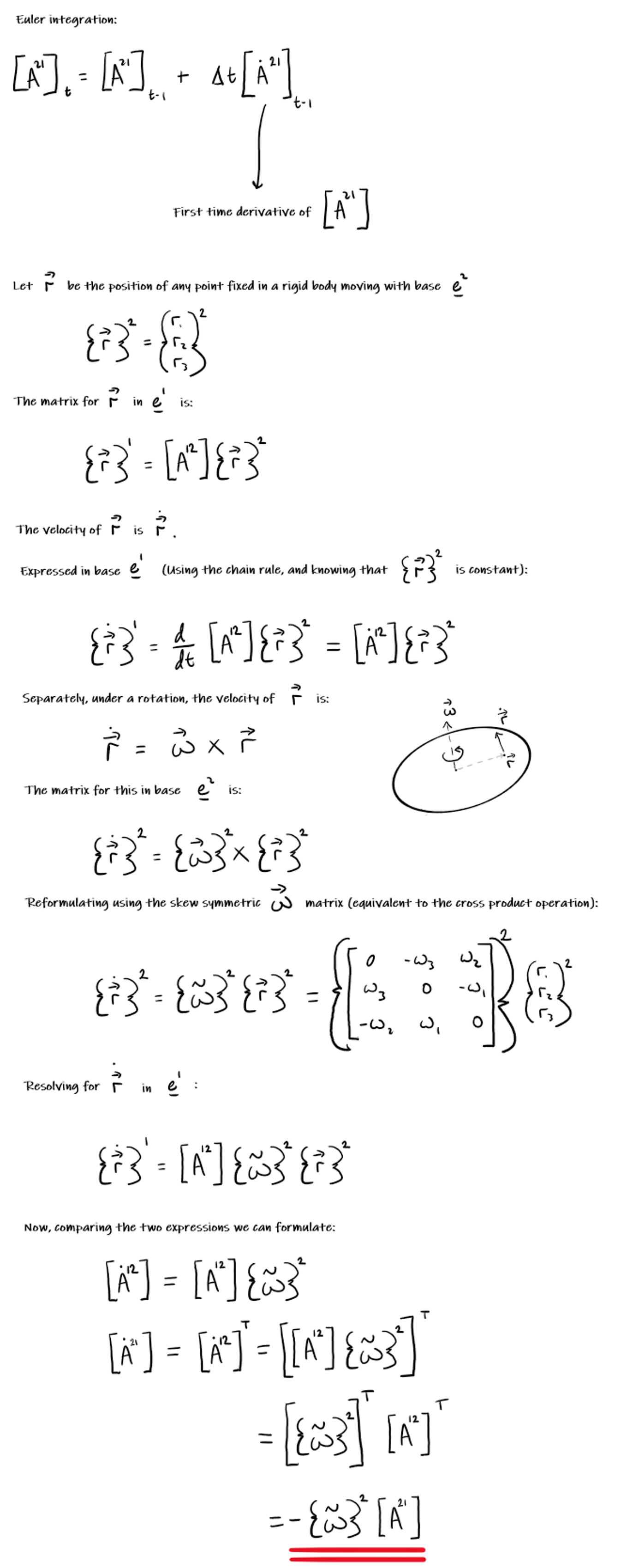The angular velocity is constant, and acts in the local coordinate system: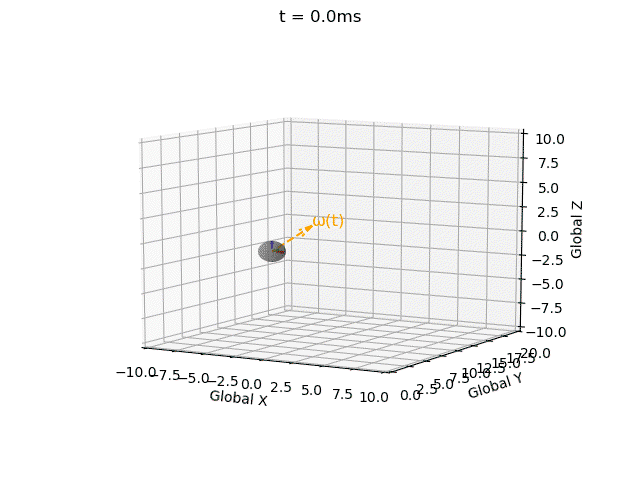We are modelling the motion of a self-propelled object, so we specify the linear velocity in the local coordinate system: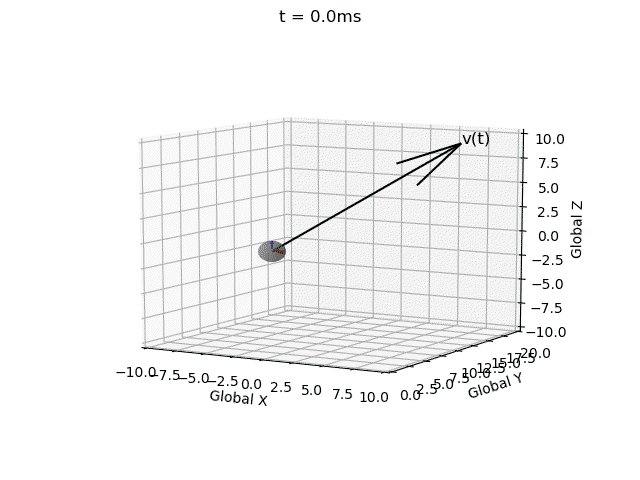### Implementation

I have impelemented Euler integration for both position and orientation of an object in a single function with intitial values, derivatives, end time, and time step as inputs (`EulerInt(A0,ω,r0,v,t_step,t_end)`).Note that for a valid rotation the rotation matrix must be 1) orthogonal and 2) have a determinant of 1. However the Euler integration approach introduces error.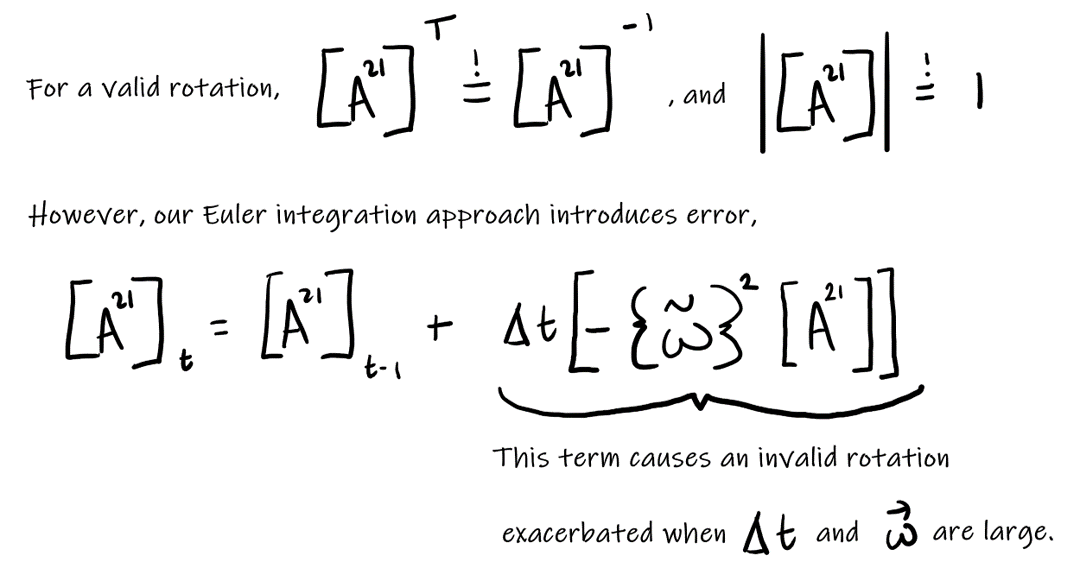Therefore I have implemented a correction term (`correction_matrix`) as described here, and a function to check validity (`isRotationMatrix(M)` where a tolerance of 1e-3 is used due to floating-point error).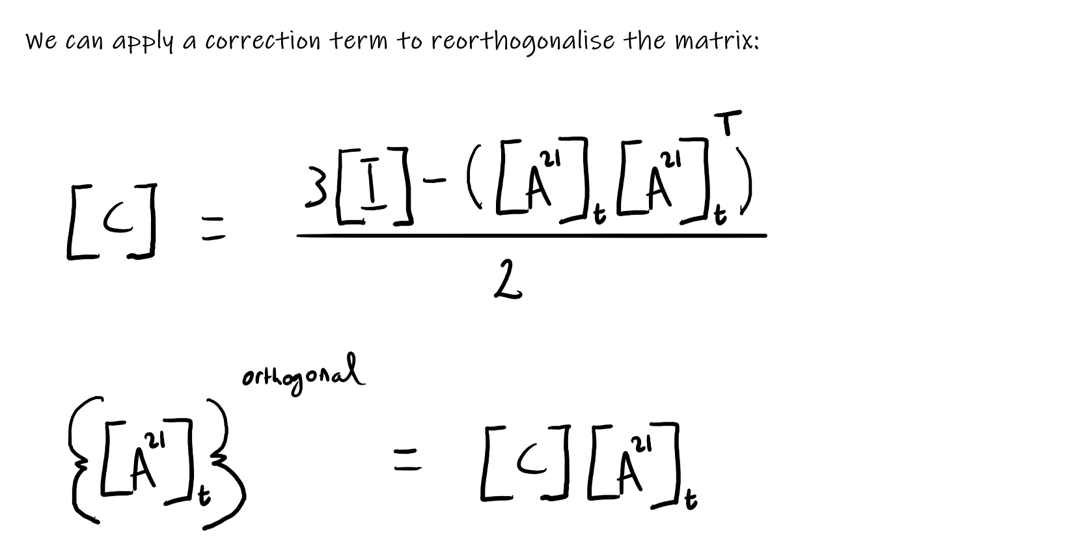Without this correction matrix the solution becomes invalid, i.e. the rotation matrix becomes non-orthogonal (indicated by the local coordinate system axes no longer being mutually perpindicular), and the determinant no longer equalling 1 (indicated by axes no longer being unit vectors). These errors propagate over time.

Without correction term: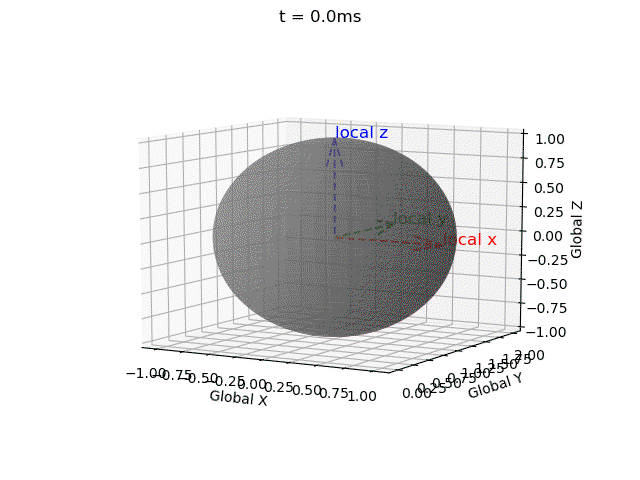With correction term: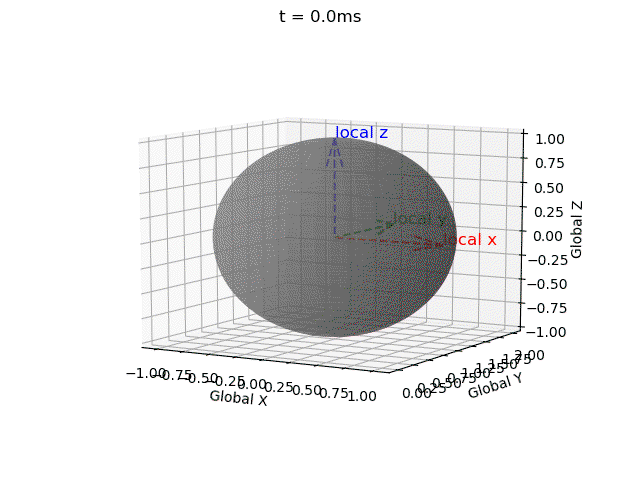The full code is available here.